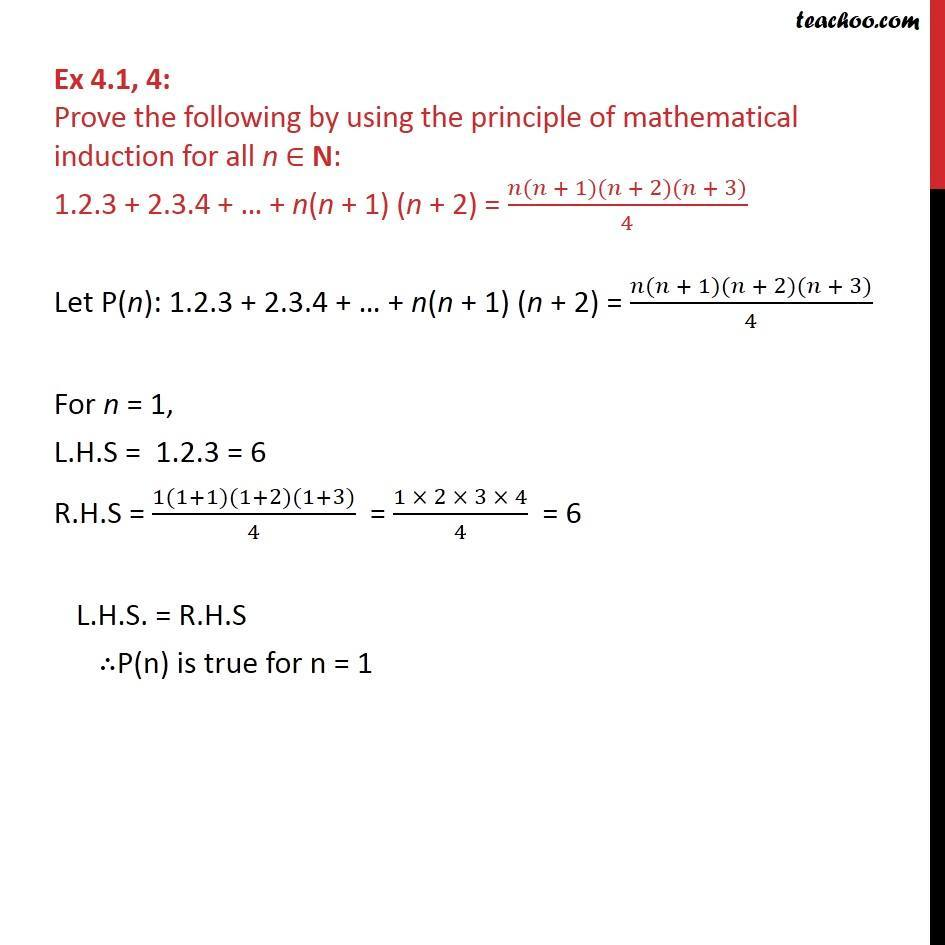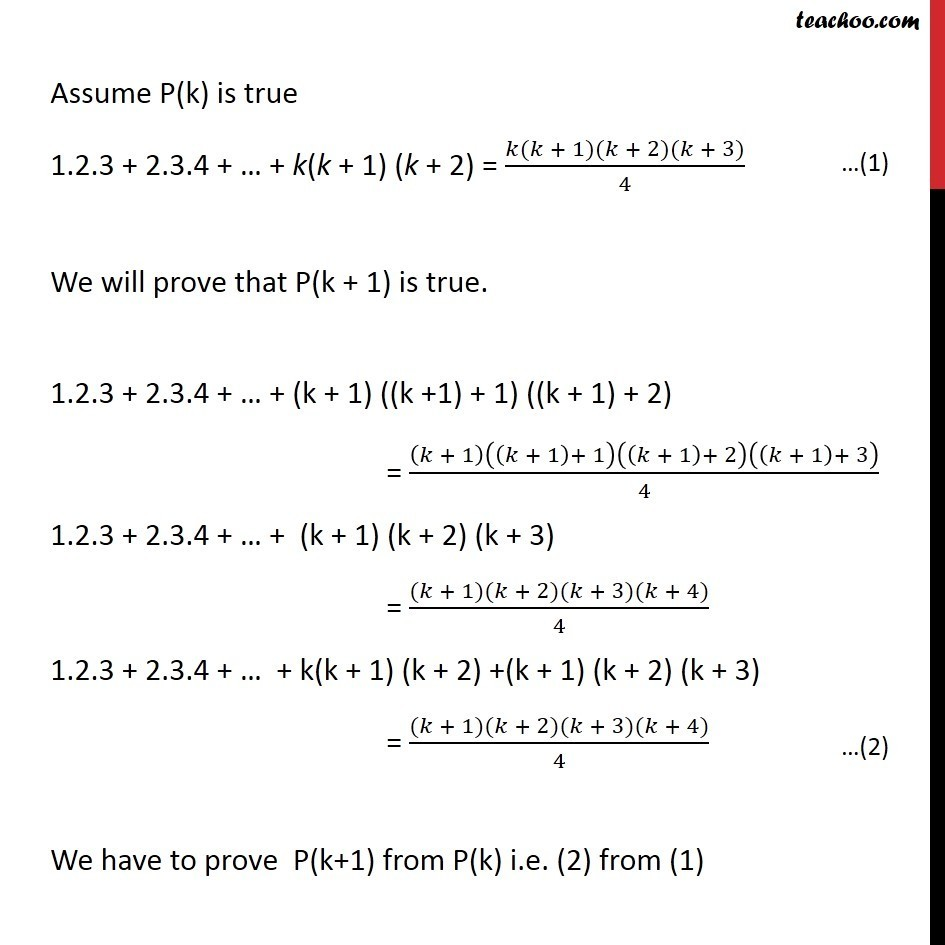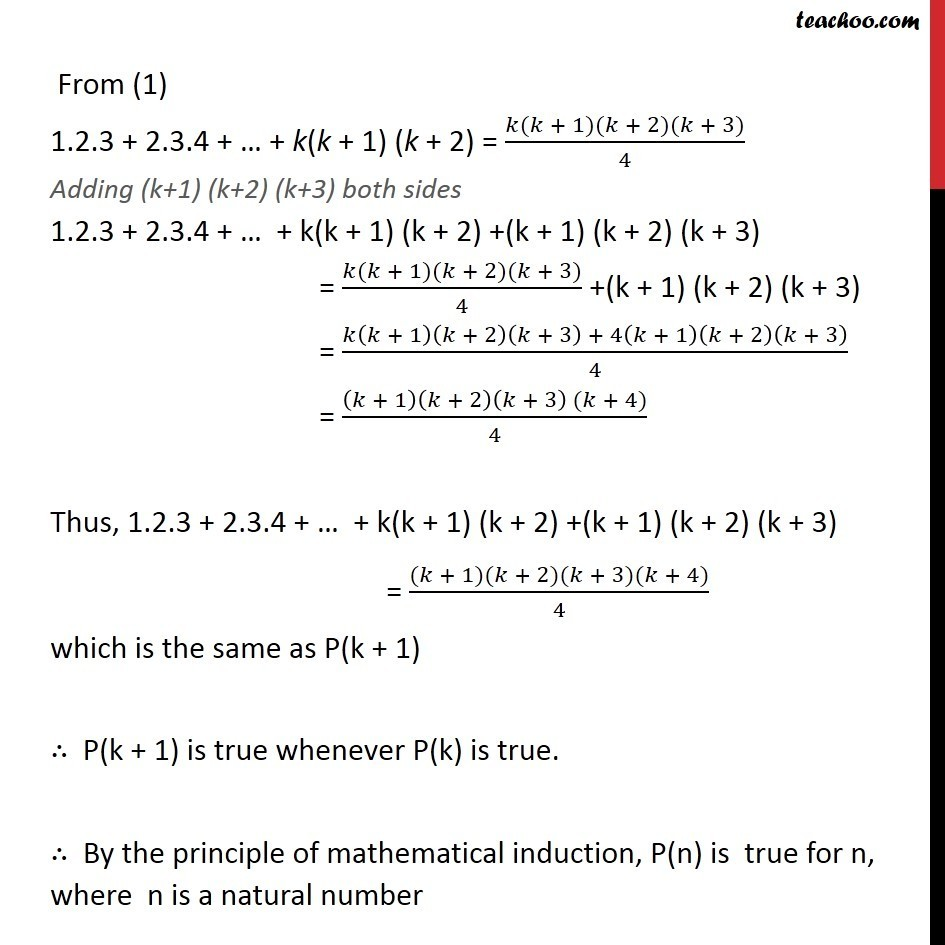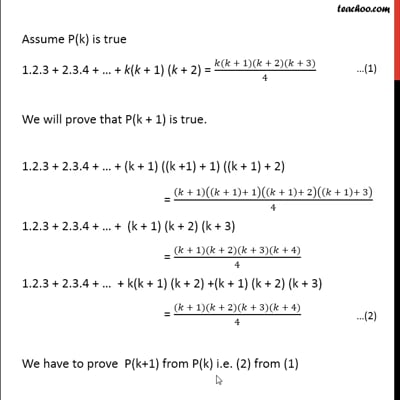Mathematical Induction - Questions and Solutions

Mathematical Induction
Serial order wiseThis video is only available for Teachoo black users

Learn in your speed, with individual attention - Teachoo Maths 1-on-1 Class

### Transcript

Question 4: Prove the following by using the principle of mathematical induction for all n ∈ N: 1.2.3 + 2.3.4 + … + n(n + 1) (n + 2) = (𝑛(𝑛 + 1)(𝑛 + 2)(𝑛 + 3))/4 Let P(n): 1.2.3 + 2.3.4 + … + n(n + 1) (n + 2) = (𝑛(𝑛 + 1)(𝑛 + 2)(𝑛 + 3))/4 For n = 1, L.H.S = 1.2.3 = 6 R.H.S = (1(1+1)(1+2)(1+3))/4 = (1 × 2 × 3 × 4)/4 = 6 L.H.S. = R.H.S ∴P(n) is true for n = 1 Assume P(k) is true 1.2.3 + 2.3.4 + … + k(k + 1) (k + 2) = (𝑘(𝑘 + 1)(𝑘 + 2)(𝑘 + 3))/4 We will prove that P(k + 1) is true. 1.2.3 + 2.3.4 + … + (k + 1) ((k +1) + 1) ((k + 1) + 2) = (𝑘 + 1)((𝑘 + 1)+ 1)((𝑘 + 1)+ 2)((𝑘 + 1)+ 3)/4 1.2.3 + 2.3.4 + … + (k + 1) (k + 2) (k + 3) = ((𝑘 + 1)(𝑘 + 2)(𝑘 + 3)(𝑘 + 4))/4 1.2.3 + 2.3.4 + … + k(k + 1) (k + 2) +(k + 1) (k + 2) (k + 3) = ((𝑘 + 1)(𝑘 + 2)(𝑘 + 3)(𝑘 + 4))/4 We have to prove P(k+1) from P(k) i.e. (2) from (1) From (1) 1.2.3 + 2.3.4 + … + k(k + 1) (k + 2) = (𝑘(𝑘 + 1)(𝑘 + 2)(𝑘 + 3))/4 Adding (k+1) (k+2) (k+3) both sides 1.2.3 + 2.3.4 + … + k(k + 1) (k + 2) +(k + 1) (k + 2) (k + 3) = (𝑘(𝑘 + 1)(𝑘 + 2)(𝑘 + 3))/4 +(k + 1) (k + 2) (k + 3) = (𝑘(𝑘 + 1)(𝑘 + 2)(𝑘 + 3) + 4(𝑘 + 1)(𝑘 + 2)(𝑘 + 3))/4 = ((𝑘 + 1)(𝑘 + 2)(𝑘 + 3) (𝑘 + 4))/4 Thus, 1.2.3 + 2.3.4 + … + k(k + 1) (k + 2) +(k + 1) (k + 2) (k + 3) = ((𝑘 + 1)(𝑘 + 2)(𝑘 + 3)(𝑘 + 4))/4 which is the same as P(k + 1) ∴ P(k + 1) is true whenever P(k) is true. ∴ By the principle of mathematical induction, P(n) is true for n, where n is a natural number